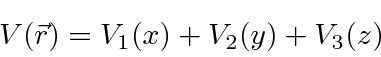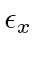# 3D Problems Separable in Cartesian Coordinates

We will now look at the case of potentials that separate in Cartesian coordinates. These will be of the form.In this case, we can solve the problem by separation of variables.The left hand side of this equation depends only on, while the right side depends onand. In order for the two sides to be equal everywhere, they must both be equal to a constantwhich we call.

Thepart of the solution satisfies the equationTreating the other components similarly we getand the total energy isThere are only a few problems which can be worked this way but they are important.

Subsections
Jim Branson 2013-04-22